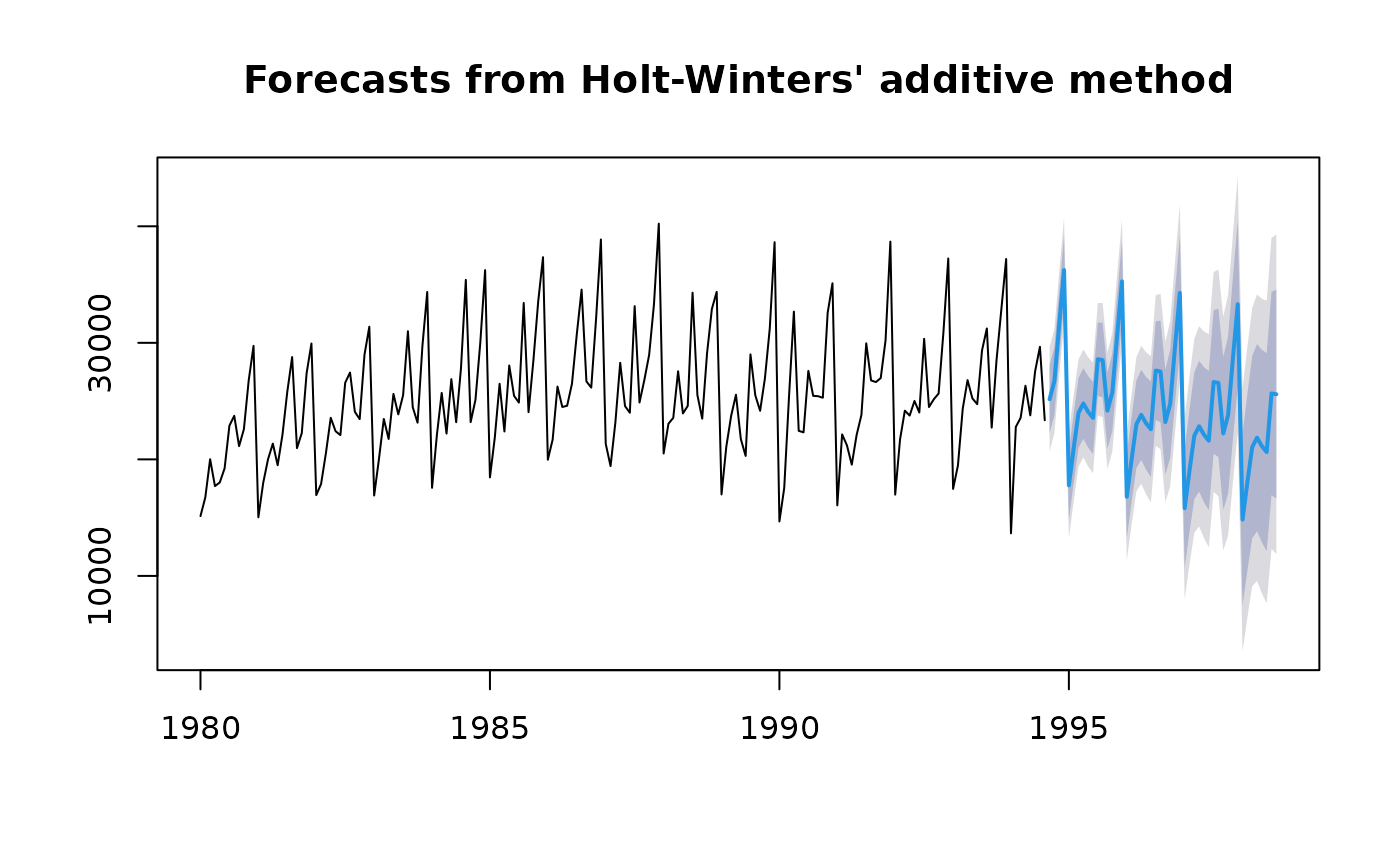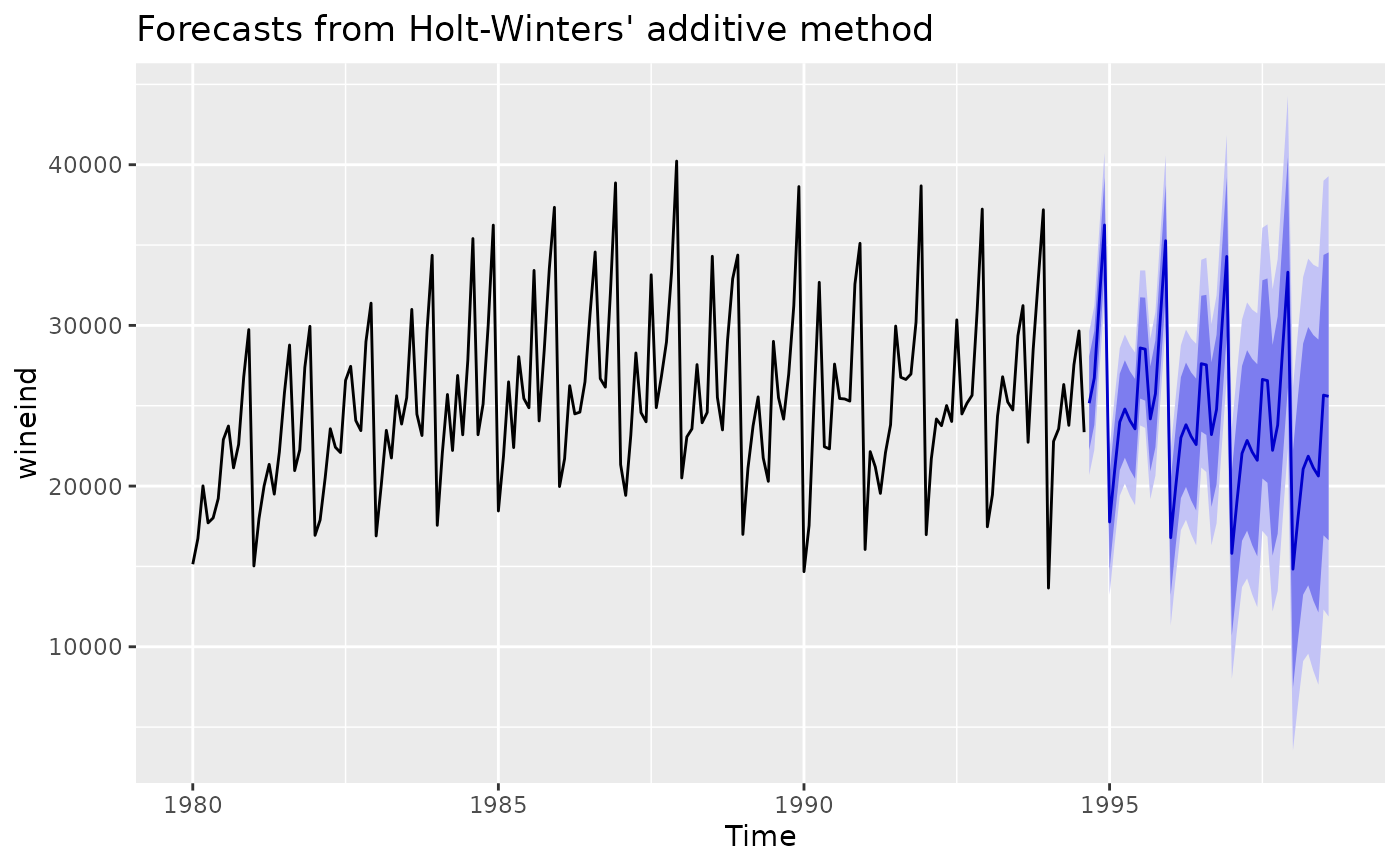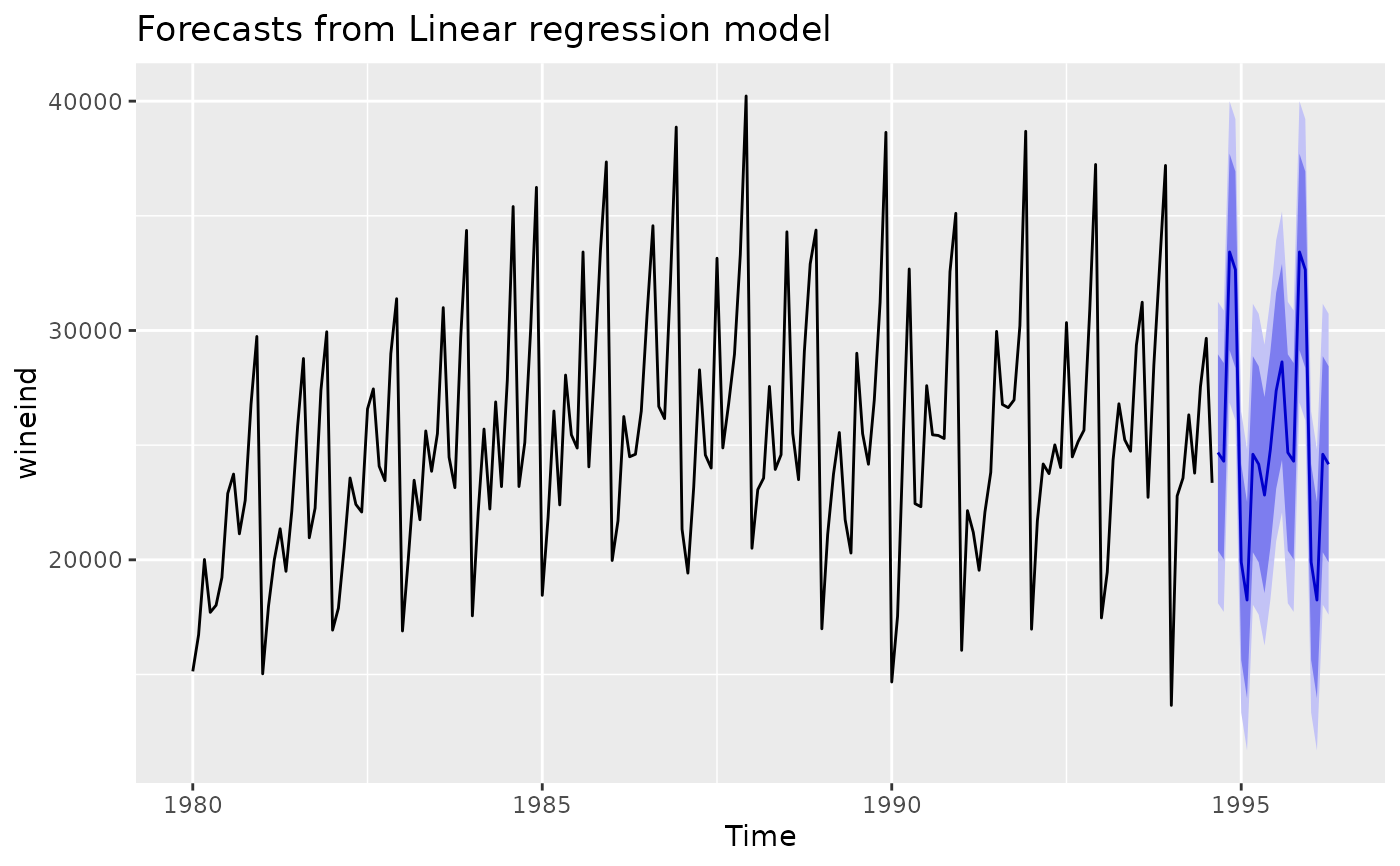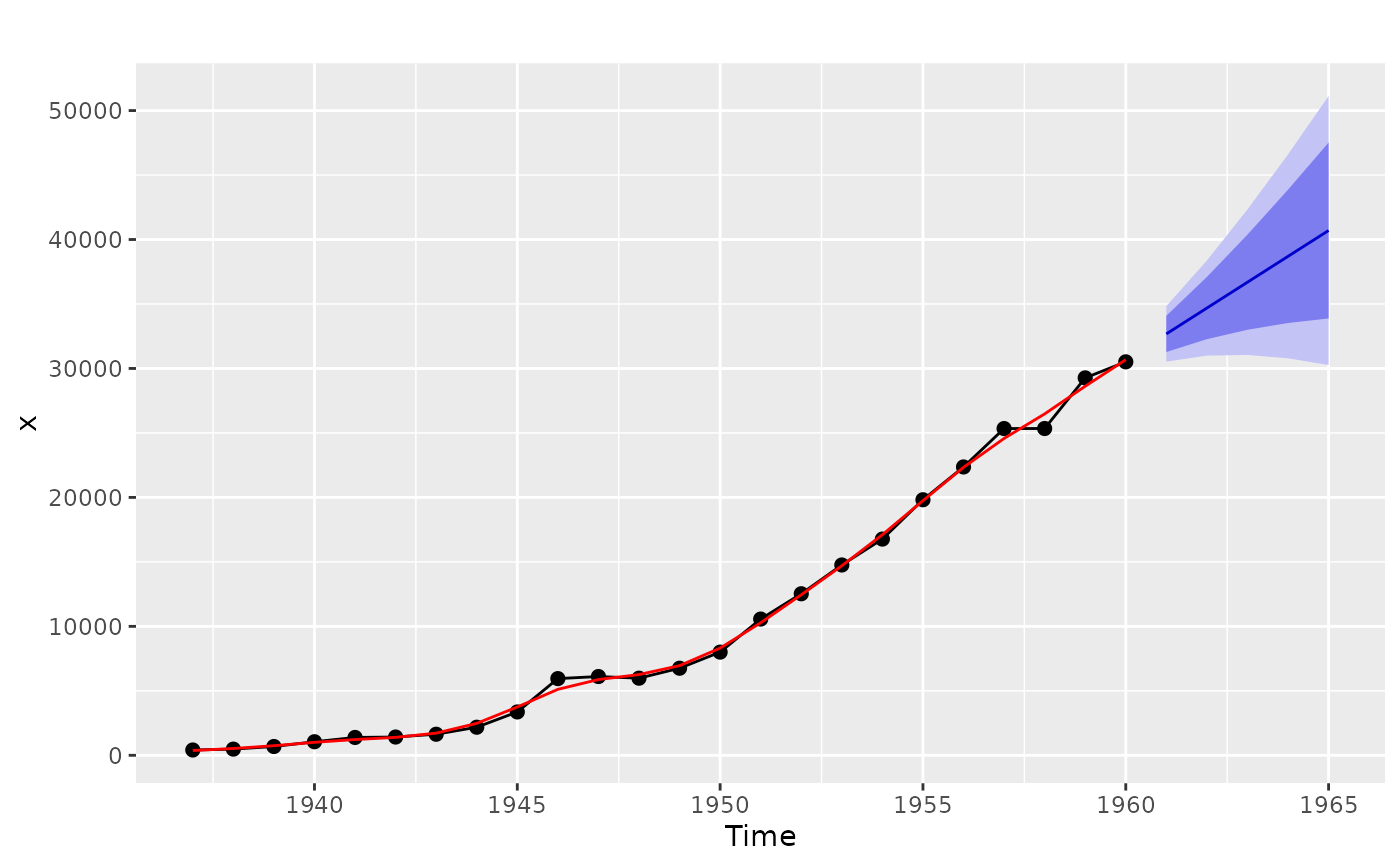Plots historical data with forecasts and prediction intervals.

# S3 method for forecast
plot(
x,
include,
PI = TRUE,
showgap = TRUE,
col = 1,
fcol = 4,
pi.col = 1,
pi.lty = 2,
ylim = NULL,
main = NULL,
xlab = "",
ylab = "",
type = "l",
flty = 1,
flwd = 2,
...
)

# S3 method for forecast
autoplot(
object,
include,
PI = TRUE,
fcol = "#0000AA",
flwd = 0.5,
...
)

# S3 method for splineforecast
autoplot(object, PI = TRUE, ...)

# S3 method for forecast
autolayer(object, series = NULL, PI = TRUE, showgap = TRUE, ...)

# S3 method for splineforecast
plot(x, fitcol = 2, type = "o", pch = 19, ...)

## Arguments

x Forecast object produced by forecast. number of values from time series to include in plot. Default is all values. Logical flag indicating whether to plot prediction intervals. If showgap=FALSE, the gap between the historical observations and the forecasts is removed. Logical flag indicating whether prediction intervals should be shaded (TRUE) or lines (FALSE) Logical flag indicating if prediction intervals should be plotted as shaded bars (if TRUE) or a shaded polygon (if FALSE). Ignored if shaded=FALSE. Bars are plotted by default if there are fewer than five forecast horizons. Colors for shaded prediction intervals. To get default colors used prior to v3.26, set shadecols="oldstyle". Colour for the data line. Colour for the forecast line. If shaded=FALSE and PI=TRUE, the prediction intervals are plotted in this colour. If shaded=FALSE and PI=TRUE, the prediction intervals are plotted using this line type. Limits on y-axis. Main title. X-axis label. Y-axis label. 1-character string giving the type of plot desired. As for plot.default. Line type for the forecast line. Line width for the forecast line. Other plotting parameters to affect the plot. Forecast object produced by forecast. Used for ggplot graphics (S3 method consistency). Matches an unidentified forecast layer with a coloured object on the plot. Line colour for fitted values. Plotting character (if type=="p" or type=="o").

None.

## Details

autoplot will produce a ggplot object.

plot.splineforecast autoplot.splineforecast

Hyndman and Athanasopoulos (2018) Forecasting: principles and practice, 2nd edition, OTexts: Melbourne, Australia. https://otexts.com/fpp2/

plot.ts

## Author

Rob J Hyndman & Mitchell O'Hara-Wild

## Examples

library(ggplot2)

wine.fit <- hw(wineind,h=48)
plot(wine.fit)autoplot(wine.fit)fit <- tslm(wineind ~ fourier(wineind,4))
fcast <- forecast(fit, newdata=data.frame(fourier(wineind,4,20)))
autoplot(fcast)fcast <- splinef(airmiles,h=5)
plot(fcast)autoplot(fcast)